# In the text we described one method for solving linear constant-coefficient difference equations,…

In the text we described one method for solving linear constant-coefficient difference equations, and another method for doing this was illustrated in Problem 2.30. If the assumption of initial rest is made so that the system described by the difference equation is LTI and causal, then, in principle, we can determine the unit impulse response h[n] using either of these procedures. In Chapter 5, we describe another method that allows us to determine h[n] in a more elegant way. In this problem we describe yet another approach, which basically shows that h[n] can be determined by solving the homogeneous equation with appropriate initial conditions. (a) Consider the system initially at rest and described by the equation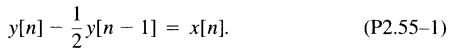Assuming that x[n] = δ[n], what is y[O]? What equation does h[n] satisfy for n ≥ 1, and with what auxiliary condition? Solve this equation to obtain a closed-form expression for h[n].

(b) Consider next the LTI system initially at rest and described by the difference equation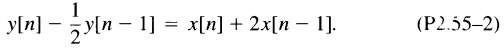This system is depicted in Figure P2.55(a) as a cascade of two LTI systems that are initially at rest. Because of the properties of LTI systems, we can reverse the order of the systems in the cascade to obtain an alternative representation of the same overall system, as illustrated in Figure P2.55(b ). From this fact, use the result of part (a) to determine the impulse response for the system described by eq. (P2.55-2)

(c) Consider again the system of part (a), with h[n] denoting its impulse response. Show, by verifying that eq. (P2.55-3) satisfies the difference equation (P2.55- 1), that the response y[ n] to an arbitrary input x[ n] is in fact given by the convolution sum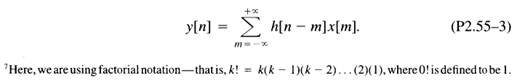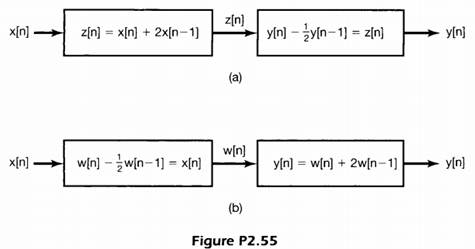(d) Consider the LTI system initially at rest and described by the difference equation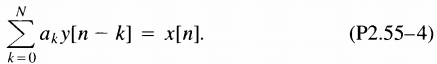Assuming that α0 ≠ 0, what is y[O] if x[n] = δ[n]? Using this result, specify the homogeneous equation and initial conditions that the impulse response of the system must satisfy.

Consider next the causal LTI system described by the difference equation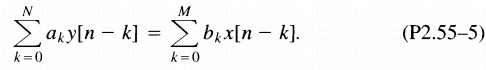Express the impulse response of this system in terms of that for the LTI system described by eq. (P2.55-4).

(e) There is an alternative method for determining the impulse response of the LTI system described by eq. (P2.55-5). Specifically, given the condition of initial rest, i.e., in this case, y[-N] = y[-N + 1] = … = y[ -1] = 0, solve eq. (P2.55-5) recursively when x[n] = δ[n] in order to determine y[O], … , y[M]. What equation does h[n] satisfy for n ≥ M? What are the appropriate initial conditions for this equation?

(f) Using either of the methods outlined in parts (d) and (e), find the impulse responses of the causal LTI systems described by the following equations: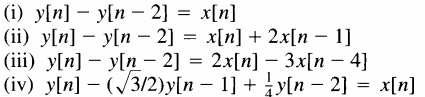Problem 2.30

Consider the first -order difference equation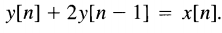Assuming the condition of initial rest (i.e., if x[n] = 0 for n 0, then y[n] = 0 for n 0), find the impulse response of a system whose input and output are related by this difference equation. You may solve the problem by rearranging the difference equation so as to express y[n] in terms of y[n -1] and x[n] and generating the values of y[O], y[ + 1 ], y[ + 2], … in that order.

#### We are the Best!##### 275 words per page

You essay will be 275 words per page. Tell your writer how many words you need, or the pages.

##### 12 pt Times New Roman

Unless otherwise stated, we use 12pt Arial/Times New Roman as the font for your paper.

##### Double line spacing

Your essay will have double spaced text. View our sample essays.

##### Any citation style

APA, MLA, Chicago/Turabian, Harvard, our writers are experts at formatting.

### We Accept

Secure Payment